/ +91 9829211106
Welcome to Vidhyarthi Darpan

# Physics 12th Previous Year Question Paper 2018 (CBSE)###### Physics 12th Previous Year Question Paper 2019 SET-II,III (CBSE)
November 29, 2019###### Maths 12th Previous Year Question Paper 2019 (CBSE)
November 30, 2019PhysicsSection – AQ.1. A proton and an electron travelling along parallel paths enters a region of uniform magnetic field, acting perpendicular to their paths. Which of them will move in a circular path with higher frequency ?

Answer: Frequency of revolution of a particle

OR

Answer: Since mass of electron is less than that of proton, therefore, its frequency of revolution will be higher than that of proton.Q.2. Name the electromagnetic radiations used for

(a) water purification, and

(b) eye surgery

(b) Eye surgery : Ultraviolet radiation/laserQ.3. Draw graphs showing variation of photoelectric current with applied voltage for two incident radiations of equal frequency and different intensities. Mark the graph for the radiation of higher intensity.

Answer : Graph for photoelectric current (I) versus applied potential for radiation of the same frequency and varying intensity.Q.4. Four nuclei of an element undergo fusion to form a heavier nucleus, with release of energy. Which of the two parent or the daughter nucleus would have higher binding energy per nucleon ?

Answer: When lighter nuclei combine to form a heavier nucleus, binding energy per nucleon increases and energy is released. Thus, the daughter nucleus would have higher binding energy per nucleon.Q.5. Which mode of propagation is used by short wave broadcast services.

Section – BQ.6. Two electric bulbs P and Q have their resistances in the ratio of 1 : 2. They are connected in series across a battery. Find the ratio of the power dissipation in these bulbs.Q.7. A 10V cell of negligible internal resistance is connected in parallel across a battery of emf 200 V and internal resistance 38 Ω as shown in the figure. Find the value of current in the circuit.

ORQ.7. In a potentiometer arrangement for deter-mining the emf of a cell, the balance point of the cell in open circuit is 350 cm. When a resistance of 9 Ω is used in the external circuit of the cell, the balance point shifts to 300 cm. Determine the internal resistance of the cell.Q.8. (a) Why are infra-red waves often called heat waves ? Explain.

(b) What do you understand by the statement, “Electromagnetic waves trans-port momentum” ?

Answer: (a) Infra-red waves are called heat waves because they raise the temperature of the object on which they fall and hence increase their thermal motion. They also affect the photographic plate and are readily absorbed by most materials.

(b) Electromagnetic waves transport momentum. This means that when an electromagnetic wave travels through space with energy U and speed c, then it transports linear momentum p = UC. If a surface absorbs the waves completely, then momentum ‘p’ is delivered to the surface. If the surface reflects the wave, then momentum delivered by both incident and reflected wave adds on to give ‘2P’ momentum.Q.9. If light of wavelength 412.5 nm is incident on each of the metals given below, which ones will show photoelectric emission and why?

Since, the energy of incident radiation is greater than the work function of sodium and potassium, but less than that of calcium and molybdenum, therefore, photoelectric emission will take place in sodium and potassium.Q.10. A carrier wave of peak voltage 15 V is used to transmit a message signal. Find the peak voltage of the modulating signal in order to have a modulation index of 60%.

Answer: Given  AC= 15v  and modulation intex μ= 60% = 60⁄100

Now, we know that

μ = AmAc

60⁄100 = Am15

Am= 15 × 0.6

Am= 9v

Hence, the peak voltage of modulating signal should be 9v in order to have a modulation index of 60%.

Section – CQ.11. Four point charges Q, q, Q and q are placed at the corners of a square of side ‘a’ as shown in the figure.

Find the

(a) resultant electric force on a charge Q and

(b) potential energy of this system.

ORQ.11. (a) Three point charges q – 4q and 2q are placed at the vertices of an equilateral triangle ABC of side ‘l’ as shown in the figure. Obtain the expression for the magnitude of the resultant electric force acting on the charge q.

(b) Find out the amount of the work done to separate the charges at infinite distance.

Answer : (a) Force on charge Q at B due to charge q at AQ.12. (a) Define the term ‘conductivity’ of a metallic wire. Write its SI unit.

(b) Using the concept of free electrons in a conductor, derive the expression for the conductivity of a wire in terms of number density and relaxation time. Hence obtain the relation between current density and the applied electric field E.

Answer: (a) Conductivity of a metallic wire is defined as its ability to allow electric charges or heat to pass through it. Numerically, conductivity of a material is reciprocal of its resistivity.

SI unit : ohm-1 m-1 or mho m-1 or Siemen m-1.

(b) Consider a potential difference V be applied across a conductor of length l and cross section A.

Electric field inside the conductor, E = v/l.

Due to the external field the free electrons inside the conductor drift with velocity Vd.

Let, number of electrons per unit volume = n,

charge on an electron = e

Total electrons in length, l = nAl And,

total charge, q = neAlQ.13. A bar magnet of magnetic moment 6 J/T is aligned at 60° with a uniform external magnetic field of 0.44 T. Calculate

(a) the work done in turning the magnet to align its magnetic moment

(i) normal to the magnetic field,

(ii) opposite to the magnetic field, and

(b) the torque on the magnet in the final orientation in case (ii).Q.14. (a) An iron ring of relative permeability μ has winding’s of insulated copper wire of n turns per meter. When the current in the winding is I, find the expression for the magnetic field in the ring.

(b) The susceptibility of a magnetic material is 0.9853. Identify the type of magnetic material. Draw the modification of the field pattern on keeping a piece of this material in a uniform magnetic field.Q.15. (a) Show using a proper diagram how unpolarised light can be linearly polarised by reflection from a transparent glass surface.

(b) The figure shows a ray of light falling normally on the face AB of an equilateral glass prism having refractive index 3/2 placed in water of refractive index 4/3 Will this ray suffer total internal reflection on striking the face AC ?Q.16. (a) If one of two identical slits producing interference in Young’s experiment is covered with glass, so that the light intensity passing through it is reduced to 50%, find the ratio of the maximum and minimum intensity of the fringes in the interference pattern.

(b) What kind of fringes do you expect to observe if white light is used instead of monochromatic light ?

Answer : (a) The resultant intensity in Young’s experiment is given by

When slit is not covered, then I0 is the intensity from each slit.

Maximum intensity (Imax) occurs when Φ = 0°.

Minimum intensity (Imin) occurs when (Φ ) = 180°.

If one slit is covered with glass to reduce its intensity by 50%, then

(b) If instead of monochromatic light, white light is used, then the central fringe will be white and the fringes on either side will be coloured. Blue colour will be nearer to central fringe and red will be farther away. The path difference at the centre on perpendicular bisector of slits will be zero for all colours and each colour produces a bright fringe thus resulting in white fringe. Further, the shortest visible wave, blue, produces a bright fringe first.Q.17. A symmetric biconvex lens of radius of curvature R and made of glass of refractive index 1.5, is placed on a layer of liquid placed on top of a plane mirror as shown in the figure. An optical needle with its tip on the principal axis of the lens is moved along the axis until its real, inverted image coincides with the needle itself. The distance of the needle from the lens is measured to be x. On removing the liquid layer and repeating the experiment, the distance is found to be y. Obtain the expression for the refractive index of the liquid in terms of x and y.

Answer: Given, refractive index of lens, μg = 1.5. The distance of the needle from the lens in the first case = The focal length of the combination of convex lens and plano concave lens formed by the liquid, f= x And, the distance measured in the second case = Focal length of the convex lens, f1 = y If the focal length of plano concave lens formed by the liquid be f2, thenQ.18. (a) State Bohr’s postulate to define stable- orbits in hydrogen atom. How does de Broglie’s hypothesis explains the stability of these orbits ?

(b) A hydrogen atom initially in the ground state absorbs a photon which excites it to the n = 4 level. Estimate the frequency of the photon.

Answer : (a) Bohr’s postulate for stable orbits in hydrogen atom : An electron can revolve only in those circular orbits in which its angular momentum is an integral multiple of h/2π, where h is Planck’s constant.

If n is the principal quantum number of orbits, then an electron can revolve only in . certain orbits or definite radii. These are called stable orbits.

de Broglie explanation of stability of orbits:

According to de Broglie, orbiting electrons around the nucleus is associated with a stationary wave. Electron wave is a circular standing wave. Since destructive interference will occur if a standing wave does not close upon itself, only those de Broglie waves exist for which the circumference of . circular orbit contains a whole number of wavelengths i.e., for orbit circumference of nth orbit as 2nπrn.

ORQ.19. (a) Explain the processes of nuclear fission and nuclear fusion by using the plot of binding energy per nucleon (BE/A) versus the mass number A.

(b) A radioactive isotope has a half-life of 10 years. How long will it take for the activity to reduce to 3.125% ?

Answer: (a) Plot of binding energy per nucleon mass number :

1. When we move from the heavy nuclei region to the middle region of the plot, we find that there will be a gain in the overall binding energy and hence results in release of energy. This indicates that energy can be released when a heavy nucleus (A ~ 240) break into two roughly equal fragments. This process is called nuclear fission.

2. Similarly, when we move from lighter nuclei to heavier nuclei, we again find that there will be gain in the overall binding energy and hence the release of energy takes place. This indicates that energy can be released when two or more lighter nuclei fuse together to form a heavy nucleus. This process is called nuclear fusion.Q.20. (a) A student wants to use two p-n junction diodes to convert alternating current into direct current. Draw a labelled circuit diagram she would use and explain how it works.

(b) Give the truth table and circuit symbol for NAND gate.

Answer : (a) Full wave rectifier :

Explanation : In positive half cycle of AC, end A becomes positive and D1 becomes forward biased and D2 is reverse biased, so conducts and D2 doesn’t. So conventional current flows through D1, RL and upper half of secondary winding. Similarly, during negative half cycle of AC, diode D2 becomes forward biased and D1 is reverse biased, current flows through D2, RL and lower half of secondary winding. Thus, current flows in the same direction in both half cycles of input AC voltage.Q.21. Draw the typical input and output characteristics of an n-p-n transistor in CE configuration. Show how these characteristics can be used to determine (a) the input resistance (r ), and (b) current amplification factor (β).

Answer: The input and output characteristics of n-p-n transistor in CE configuration is given below:

Input resistance is defined as the ratio of change in base-emitter voltage (ΔVBE) to the change in base current (ΔIB) at a constant collector-emitter voltage.

Current amplification factor (β) is defined as the ratio of the change in the collector current to the change in the base current at a constant collector-emitter voltage when the transistor is in active state.Q.22. (a) Give three reasons why modulation of a message signal is necessary for long distance transmission.

(b) Show graphically an audio signal, a carrier wave and an amplitude modulated wave.

(a) The three reasons necessary for long-distance transmission are:

i) A reasonable length of the transmission antenna.

ii) Increase in effective power radiated by the antenna.

iii) Reduction in the possibility of “mix-up‟ of different signals.

(b) In Amplitude modulation, the transmission of a wave signal is done by modulating the amplitude. At the source, the information signal is modulated and sent to the receiver, once it is received, it is then demodulated and filtered to remove any disturbance in the original signal that may have occurred during the transmission. The amplitude modulation is the process of superimposing the information signal with a carrier generated signal.

The information signal is the data message signal stored on a network that has to be transmitted from a source to a receiver.A carrier wave is an electromagnetic wave of fixed amplitude and of a constant frequency that is modulated with an input message signal for the purpose of transmission.

Section – DQ.23. The teachers of Geeta’s school took the students on a study trip to a power generating station, located nearly 200 km away from the city. The teacher explained that electrical energy is transmitted over such a long distance to their city, in the form of alternating current (ac) raised to a high voltage. At the receiving end in the city, the voltage is reduced to operate the devices. As a result, the power loss is reduced. Geeta listened to the teacher and asked questions about how the ac is converted to a higher or lower voltage.

(a) Name the device used to change the alternating voltage to a higher or lower value. State one cause for power dissipation in this device.

(b) Explain with an example, how power loss is reduced if the energy is transmitted over long distances as an alternating current rather than a direct current.

(c) Write two values each shown by the teachers and Geeta.

Answer : (a) The device used to change the alternating voltage to a higher or lower value is step up or step-down transformer. It works on the principle of Faraday’s law of induction by converting one value of electrical energy into another.

One of the reasons for power dissipation in this device is the production of eddy currents in core. The changing magnetic field induces an EMF in the secondary winding. However, due to this changing magnetic field through the core, an EMF is induced in the core itself as the core is made of conducting material and thus current is generated in the core. These circulating currents are in the form of swirling eddies, called eddy currents.

(b) The relation between the voltage in the secondary coil with a voltage in the primary coil is given as

Vs = (Ns/Np) Vp

And the relation between current in the secondary coil with a current in the primary coil is given as

Is =(Np/Ns) Ip

In a step-up transformer, if the voltage is getting stepped up, then current in the secondary coil is getting reduced.

Hence the power loss (P = I2R) is reduced considerably while such stepping up is not possible for direct current.

(c) The teacher is well informed and willing to share her knowledge with Geeta that reflects her concerns for her. She wants her students to learn the practical aspects of the theoretical learning that is done in the class.

Geeta, on the other hand, manifest the value of a good listener who is interested in learning the practical application of the concepts that has been taught in the class. It reflects her curious nature and the sheer willingness to learn something new.

Section – EQ.24. (a) Define electric flux. Is it a scalar or a vector quantity ? A point charge q is at a distance of dl2 directly above the center of a square of side d, as shown in the figure. Use Gauss’ law to obtain an expression for the electric flux through the square.

(b) If the point charge is now moved to a distance ‘d ‘ from the center of the square and the side of the square is doubled, explain how the electric flux will be affected.

ORQ.24. (a) Use Gauss’ law to derive the expression for the electric field (E) due to a straight uniformly charged infinite line of charge density λ C/m.

(b) Draw a graph to show the variation of E with perpendicular distance r from the line of charge.

(c) Find the work done in bringing a charge q from perpendicular distance r1to r2( r2 > r1).

Answer: (a) Electric flux : Electric flux through an area is defined as the product of electric field strength E and area dS perpendicular to the field. It represents the field lines crossing the area. It is a scalar quantity. Imagine a cube of edge d, enclosing the charge. The square surface is one of the six faces of this cube. According to Gauss’ theorem in electrostatics,

Total electric flux through the cube =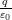This is the total flux through all six surface

∴ Electric flux through the square surface =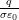(b) On moving the charge to distance d from the center of the square and making side of square 2d, does not change the flux at all because flux is independent of side of square or distance of charge in this case.

OR

Answer: (a) Electric field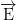due to a straight uniformly charged infinite line of charge density λ : Consider a cylindrical Gaussian surface of radius r and length l coaxial with line charge. The cylindrical Gaussian surface may be divided into three parts :

(i) curved surface S1

(ii) flat surface S2 and

(iii) flat surface S3.

By symmetry, the electric field has the same magnitude E at each point of curved surface Sj and is directed radially outward. We consider small elements of surfaces S1, S2 and S3.

b) Graph showing variation of E with perpendicular distance from the line of charge : The electric field is inversely proportional to distance V from line of charge.Q.25. (a) State the principle of an ac generator and explain its working with the help of a labelled diagram. Obtain the expression for the emf induced in a coil having N turns each of cross-sectional area A, rotating with a constant angular speed ω in a magnetic field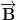, directed perpendicular to the axis of rotation.

(b) An aeroplane is flying horizontally from west to east with a velocity of 900 km/ hour. Calculate the potential difference developed between the ends of its wings having a span of 20 m. The horizontal component of the Earth’s magnetic field is 5 × 10-4T and the angle of dip is 30°.

ORQ.25. A device X is connected across an ac source of voltage V = V0sin ωt . The current through X is given as I = I0sin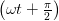(a) Identify the device X and write the expression for its reactance.

(b) Draw graphs showing variation of voltage and current with time over one cycle of ac, for X.

(c) How does the reactance of the device X varies with frequency of the ac ? Show this variation graphically.

(d) Draw the phasor diagram for the device X.

Answer : (a) Principle of ac generator :

The ac generator is based on the principle of electromagnetic induction. When closed coil is rotated in a uniform field with its axis perpendicular to the field, then the magnetic flux changes and emf is induced.

Working : When the armature coil rotates, the magnetic flux linked with it changes and produces induced current. If initially, coil PQRS is in vertical position and rotated clockwise, then PQ moves down and SR moves up. By Fleming’s right hand rule, induced current flows from Q to P and S to R which is the first half rotation of coil. Brush B1is positive terminal and B2 is negative. In second half rotation, PQ moves up and SR moves down. So induced current reverses and the alternating current is produced in this manner by the generator.Q.26. (a) Draw a ray diagram to show image formation when the concave mirror produces a real, inverted and magnified image of the object.

(b) Obtain the mirror formula and write an expression for the linear magnification.

(c) Explain two advantages of a reflecting telescope over a refracting telescope.

ORQ.26. (a) Define a wave front. Using Huygens’ principle, verify the laws of reflection at a plane surface.

(b) In a single slit diffraction experiment, the width of the slit is made double the original width. How does this affect the size and intensity of the central diffraction band ? Explain.

(c) When a tiny circular obstacle is placed in the path of light from a distant source, a bright spot is seen at the center of the obstacle. Explain why ?

Answer : (a) Concave mirror produces real, inverted and magnified image for object placed between F and C :

(b) Derivation for mirror formula and magnification: Consider an object AB be placed in front of a concave mirror beyond center of curvature C.

(c) Advantages of reflecting telescope over a refracting telescope are :

1. Reflecting telescope is free from chromatic and spherical aberrations unlike refracting telescope. Thus image formed is sharp and bright.

2. It has a larger light gathering power so that a bright image of even far off object is obtained.

3. Resolving power of reflecting telescope is large.

OR

Answer: (a) Wave front is defined as the continuous locus of all the particles of a medium which are vibrating in the same phase. Verification of laws of reflection using Huygens’s principle : Let XY be a reflecting surface at which a wave front is being incident obliquely. Let v be the speed of the wave front and at time t = 0, the wave front touches the surface XY at A. After time t, point B of wave front reaches the point B’ of the surface.

According to Huygens’s principle each point of wave front acts as a source of secondary waves. When the point A of wave front strikes the reflecting surface, then due to the presence of reflecting surface, it cannot advance further; but the secondary wavelet originating from point A begins to spread in all directions in the first medium with speed v. As the wave front AB advances further, its points A1, A2, A3 etc. strike the reflecting surface successively and send spherical secondary wavelets in the first medium.